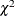The MCMC Procedure

Using Density Functions in the Programming Statements

Subsections:

Density Functions in PROC MCMC

PROC MCMC has a number of internally defined log-density functions for univariate and multivariate distributions. These functions have the basic form of LPDFdist(x, parm-list), where dist is the name of the distribution (see Table 73.41 for univariate distributions and Table 73.42 for multivariate distributions). The argument x is the random variable, and parm-list is the list of parameters.

In addition, the univariate functions allow for optional boundary arguments, such as LPDFdist(x, parm-list, <lower>, <upper>), where lower and upper are optional but positional boundary arguments. With the exception of the Bernoulli and uniform distribution, you can specify limits on all univariate distributions.

To set a lower bound on the normal density:

lpdfnorm(x, 0, 1, -2);


To set just an upper bound, specify a missing value for the lower bound argument:

lpdfnorm(x, 0, 1, ., 2);


Leaving both limits out gives you the unbounded density. You can also specify both bounds:

lpdfnorm(x, 0, 1);
lpdfnorm(x, 0, 1, -3, 4);


See Table 73.41 for the function names of univariate distributions and Table 73.42 for multivariate distributions.

Table 73.41: Logarithm of Univariate Density Functions in PROC MCMC

Distribution Name

Function Call

Beta

lpdfbeta (x, a, b,<lower>, <upper>);

Binary

lpdfbern (x, p);

Binomial

lpdfbin (x, n,p, <lower>, <upper>);

Cauchy

lpdfcau (x, loc, scale, <lower>, <upper>);lpdfchisq (x, df,<lower>, <upper>);

Exponentiallpdfechisq (x, df, <lower>, <upper>);

Exponential gamma

lpdfegamma (x, sp,scale, <lower>, <upper>);

Exponential exponential

lpdfeexpon (x, scale,<lower>, <upper>);

Exponential inverselpdfeichisq (x, df, <lower>, <upper>);

Exponential inverse-gamma

lpdfeigamma (x, sp, scale, <lower>, <upper>);

Exponential scaled inverselpdfesichisq (x, df, scale, <lower>, <upper>);

Exponential

lpdfexpon (x, scale, <lower>, <upper>);

Gamma

lpdfgamma (x, sp, scale, <lower>, <upper>);

Geometric

lpdfgeo (x, p, <lower>, <upper>);

Inverselpdfichisq (x, df, <lower>, <upper>);

Inverse-gamma

lpdfigamma (x, sp, scale, <lower>, <upper>);

Laplace

lpdfdexp (x, loc, scale, <lower>, <upper>);

Logistic

lpdflogis (x, loc, scale, <lower>, <upper>);

Lognormal

lpdflnorm (x, loc, sd, <lower>, <upper>);

Negative binomial

lpdfnegbin (x, n, p, <lower>, <upper>);

Normal

lpdfnorm (x, mu, sd, <lower>, <upper>);

Pareto

lpdfpareto (x, sp, scale, <lower>, <upper>);

Poisson

lpdfpoi (x, mean, <lower>, <upper>);

Scaled inverselpdfsichisq (x, df, scale, <lower>, <upper>);

t

lpdft (x, mu, sd, df, <lower>,<upper>);

Uniform

lpdfunif (x, a, b);

Wald

lpdfwald (x, mean, scale, <lower>, <upper>);

Weibull

lpdfwei (x, loc, sp, scale, <lower>, <upper>);

In the multivariate log-density functions, arrays must be used in place for the random variable and parameters in the model.

Table 73.42: Logarithm of Multivariate Density Functions in PROC MCMC

Distribution Name

Function Call

Dirichlet

lpdfdirich (x_array, alpha_array);

Inverse Wishart

lpdfiwish (x_array, df, S_array);

Multivariate normal

lpdfmvn (x_array, mu_array, cov_array);

Multinomial

lpdfmnom (x_array, p_array);

Standard Distributions, the LOGPDF Functions, and the LPDFdist Functions

Standard distributions listed in the section Standard Distributions are names only, and they can be used only in the MODEL , PRIOR , and HYPERPRIOR statements to specify either a prior distribution or a conditional distribution of the data given parameters. They do not return any values, and you cannot use them in the programming statements.

The LOGPDF functions are DATA step functions that compute the logarithm of various probability density (mass) functions. For example,
logpdf ("beta", x, 2, 15); returns the log of a beta density with parameters a = 2 and b = 15, evaluated at x. All the LOGPDF functions are supported in PROC MCMC.

The LPDFdist functions are unique to PROC MCMC. They compute the logarithm of various probability density (mass) functions. The functions are the same as the LOGPDF functions when it comes to calculating the log density. For example,
lpdfbeta (x, 2,15); returns the same value as
logpdf ("beta", x, 2, 15); The LPDFdist functions cover a greater class of probability density functions, and the univariate distribution functions take the optional but positional boundary arguments. There are no corresponding LCDFdist or LSDFdist functions in PROC MCMC. To work with the cumulative probability function or the survival functions, you need to use the LOGCDF and the LOGSDF DATA step functions.